# Java开发笔记（一百四十五）FXML布局的伸展适配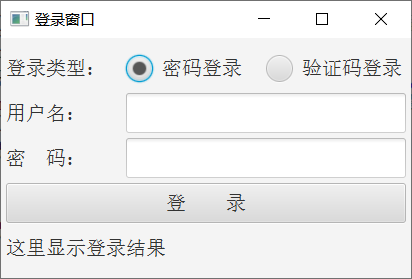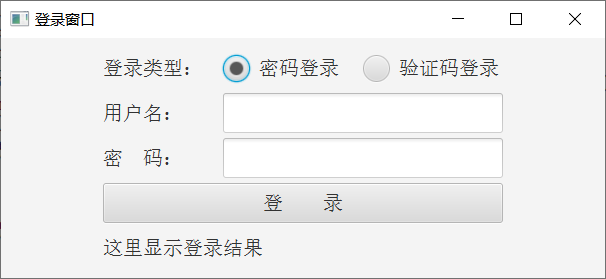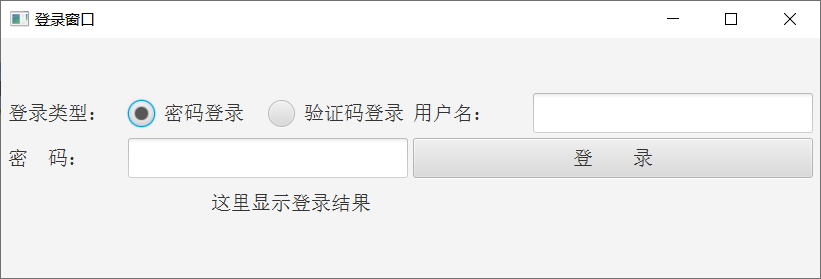<VBox fx:controller="com.javafx.fxml.LoginController" xmlns:fx="http://javafx.com/fxml" alignment="center">
<!-- 这是xml注释标记。中间省略登录窗口的各控件标签 -->
</VBox>


fxml文件修改完毕，重新运行测试程序，弹出的登录窗口如下图所示。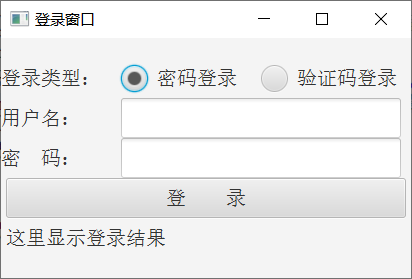1、登录类型、用户名、密码三块区域的左侧直接顶到了窗口边缘；
2、用户名输入框、密码输入框、登录按钮三个自上往下紧紧贴着，不留一丝空隙；

  <padding>
<Insets top="10.0" bottom="10.0" left="10.0" right="10.0"/>


<VBox fx:controller="com.javafx.fxml.LoginController" xmlns:fx="http://javafx.com/fxml" alignment="center">
<children>
<!-- 这是xml注释标记。中间省略VBox的下级控件列表 -->
</children>
<Insets top="10.0" bottom="10.0" left="10.0" right="10.0"/>
</VBox>


<VBox fx:controller="com.javafx.fxml.LoginController" xmlns:fx="http://javafx.com/fxml" alignment="center">
<children>
<HBox fx:id="hbType" prefWidth="400" prefHeight="40">
<children>
<Label fx:id="labelType" prefWidth="120" prefHeight="40" text="登录类型：" />
<fx:define>
<ToggleGroup fx:id="tgType" />
</fx:define>
<RadioButton fx:id="rbPassword" prefWidth="140" prefHeight="40" toggleGroup="$tgType" text="密码登录" selected="true" /> <RadioButton fx:id="rbVerifycode" prefWidth="140" prefHeight="40" toggleGroup="$tgType"
text="验证码登录" />
</children>
<Insets top="0.0" bottom="10.0" left="0.0" right="0.0"/>
</HBox>
<HBox fx:id="hbUser" prefWidth="400" prefHeight="40" VBox.vgrow="ALWAYS">
<children>
<Label fx:id="labelUser" prefWidth="120" prefHeight="40" text="用户名：" />
<TextField fx:id="fieldUser" prefWidth="280" prefHeight="40" HBox.hgrow="ALWAYS" />
</children>
<Insets top="0.0" bottom="10.0" left="0.0" right="0.0"/>
</HBox>
<children>
<Label fx:id="labelPassword" prefWidth="120" prefHeight="40" text="密　码：" />
</children>
<Insets top="0.0" bottom="10.0" left="0.0" right="0.0"/>
</HBox>
<Button fx:id="btnLogin" prefWidth="400" prefHeight="40" text="登　　录" />
<Label fx:id="labelLoginResult" prefWidth="400" prefHeight="40" text="这里显示登录结果" />
</children>
<Insets top="10.0" bottom="10.0" left="10.0" right="10.0"/>
</VBox>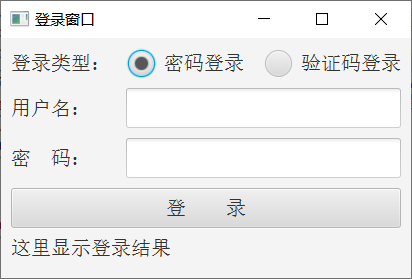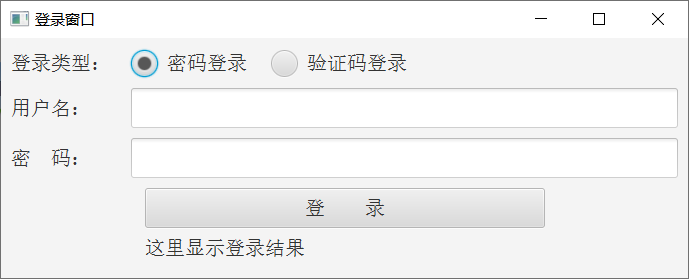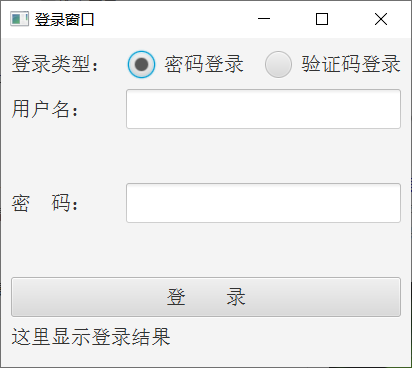posted @ 2019-09-02 19:03  pinlantu  阅读(1191)  评论(0编辑  收藏  举报